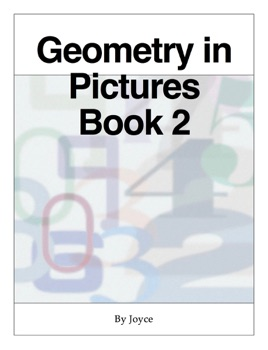## Description de l’éditeur

Geometry in Pictures Book 2 is the seventh book in the series, covering the sine, cosine and tangent ratios, calculating unknown lengths and angles in right angles triangles. The exact values of the trigonometry ratios for 30, 45 and 60 degrees, and relationships between the trigonometry functions. Bearings are defined, and trigonometry and Pythagoras’ theorem applied to calculate angles and distances. Formulae are used for the circumference and area of a circle, the length of arc and area of a sector, perimeters, and areas of figures including minor arcs and segments of circles. Drawing 3D figures using oblique projection, nets, Euler’s relation and the symmetries of polyhedra. The surface area and volume of the cuboid, prism & pyramid, the cylinder, cone & hemisphere. There are galleries and review questions.

The review questions require iOS 10 or higher.